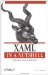# Matrix

 Matrix Matrix is a structure and has no class hierarchy.

`         <Matrix             M11="2.0"             M12="3.0"             M21="2.0"             M22="3.0"             OffsetX="1.0"             OffsetY="1.0" /> `

<Matrix .../> represents a 3 x 3 matrix used for transformations. Avalon supports only affine transformations linear transformations followed by a translationso the matrix has only six entries instead of nine. The final three entries in the matrix are predefined, as shown in Table 11-1.

M11

M12

0

M21

M22

0

M11

M12

0

#### Attributes

M11 (required)

A Double value representing the value in the first row and first column of the Matrix

M12 (required)

A Double value representing the value in the first row and second column of the Matrix

M21 (required)

A Double value representing the value in the second row and first column of the Matrix

M22 (required)

A Double value representing the value in the second row and second column of the Matrix

OffsetX (optional)

A Double value representing the amount of the x-offset in the translation following the transformation

OffsetY (optional)

A Double value representing the amount of the y-offset in the translation following the transformationXAML in a Nutshell (In a Nutshell (OReilly))
ISBN: 0596526733
EAN: 2147483647
Year: 2007
Pages: 217